# XLOOKUP by Date – Excel & Google Sheets

This tutorial will demonstrate how to use the XLOOKUP Function with dates in Excel.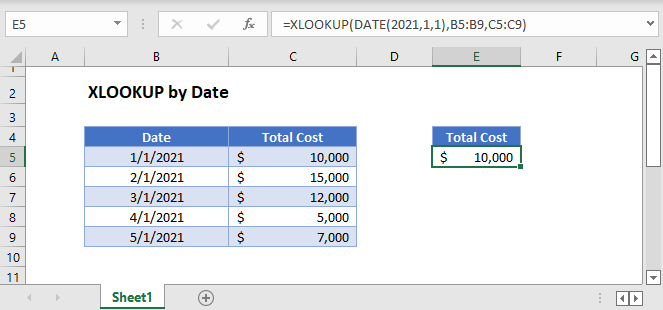In Excel, dates are stored as serial numbers where each number represents a unique date (the number of days from the imaginary day 1/0/1900).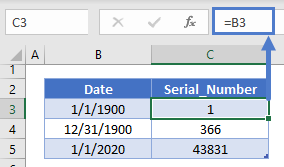Later in this tutorial we will discuss how to deal with dates stored as text, but for now, we will assume all dates are stored as serial numbers.

### XLOOKUP with Date Function

One way to enter a date into an XLOOKUP formula is to use the DATE Function.

``=XLOOKUP(DATE(2021,1,1),B3:B7,C3:C7)``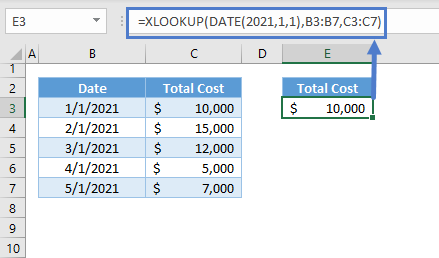The DATE Function generates a date from a given year, month, and day.

### XLOOKUP with DATEVALUE Function

Another method to create a date is to use the DATEVALUE Function.

``=XLOOKUP(DATEVALUE("1/1/2021"),B3:B7,C3:C7)``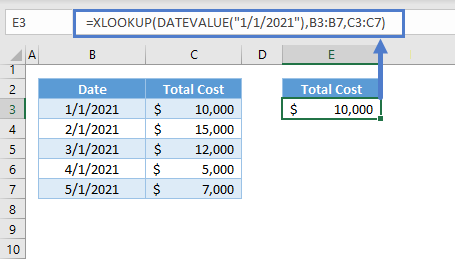The DATEVALUE Function converts a string of text that represents a date, into a date that Excel can work with (a serial number date).

## XLOOKUP with Dates stored in Cells

Alternatively, you can reference a cell containing a date to perform an XLOOKUP.

``=XLOOKUP(E3,B3:B7,C3:C7)``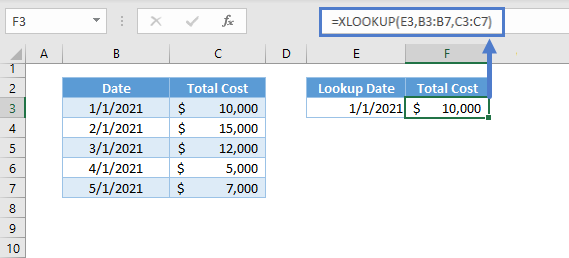## XLOOKUP with Multiple Dates

We can reference a list of lookup dates, which will convert the formula to a dynamic array formula.

``=XLOOKUP(E3:E4,B3:B7,C3:C7)``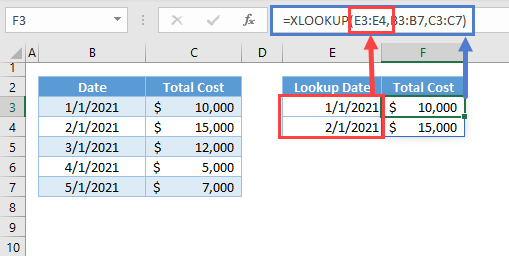Note: When a list of values is inputted in an argument that normally can only accept a single value, a dynamic array or Spill Formula is created. The formula will “spill” to perform the calculations on the entire array of values.

## XLOOKUP with Approximate Match

By default, the XLOOKUP will perform an exact match. Changing match_mode (5th argument) to -1 or 1 will search for an inexact match instead.

### XLOOKUP with Next Smaller Item

We can search for the exact date or the latest date before the lookup date by changing the match_mode to -1.

``=XLOOKUP(E3,B3:B7,C3:C7,,-1)``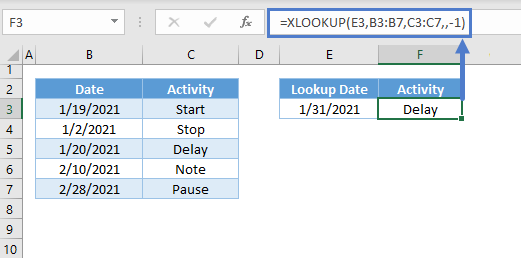Note: If the XLOOKUP can’t find the exact match, it will return the largest value that is less than the lookup value.

### XLOOKUP with Next Larger Item

If we want to return an approximate value that is greater than the lookup value, then we need to apply match_mode = 1.

``=XLOOKUP(E3,B3:B7,C3:C7,,1)``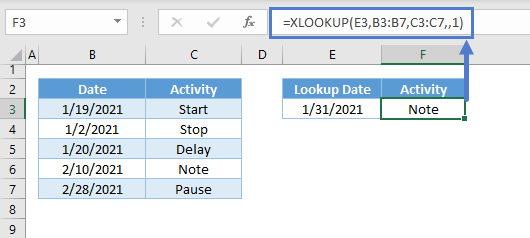Note: If the XLOOKUP Function can’t find the exact match, it will find the smallest value that is greater than the lookup value.

## XLOOKUP with Last Date

By default, the XLOOKUP Function will start searching top to bottom. Changing search_mode (6th or last argument) to -1 will change the search order to Bottom-up.

We can search for the last occurrence of the lookup date within the list by inputting -1 in the last argument.

``=XLOOKUP(E3,B3:B7,C3:C7,,1,-1)``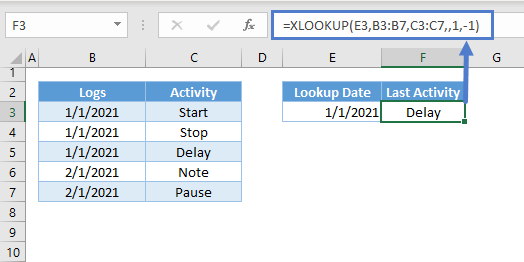## XLOOKUP with Date-Time Data Type

As noted above, Excel stores dates as whole serial numbers. Time is stored as a decimal value representing the fraction of the day.

To get the serial number only, we can use the INT Function.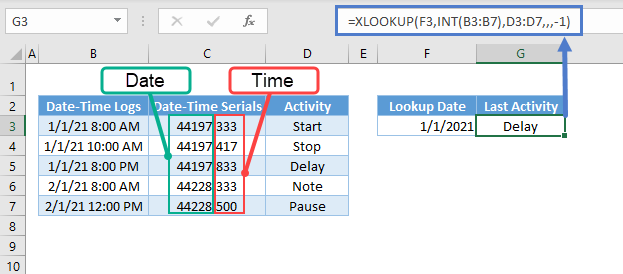The INT Function returns only the integer (date) portion of a number.

## XLOOKUP Date Problems

Usually issues with Date lookups, stem from issues with the data type of the raw data (e.g., text, number, date). One important rule for the XLOOKUP Function is that the data type of the lookup value must be the same with the data type of the values within the lookup list. If the dates in the data set are in text and the lookup value is in date, then the XLOOKUP Function will return the #N/A error.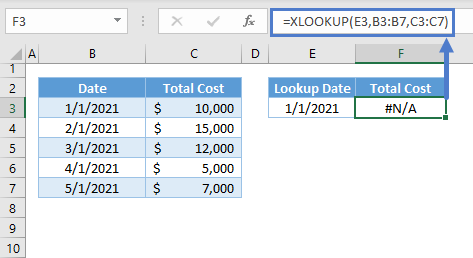### Dates as Texts

The fastest way to check if the dates are in text is to look at the alignment of the data. If it’s left aligned, then it’s a text, and if it’s right aligned, then it’s a number.

Another way is to look at the number formatting. Just go to Home > Number > Number Format and check if the formatting is Text.

To convert the number stored as text to a number, you can use the DATEVALUE Function or another one of the options discussed in the linked tutorial.

### Imported or Copied Dates

Data that are imported or copied from other sources (e.g., web, csv) will not be in date data type most of the time. To fix this problem, we can use the Text to Columns Tool of Excel to fix our date problem.

Steps:

1. Highlight the dates and go to Data > Data Tools > Text to Columns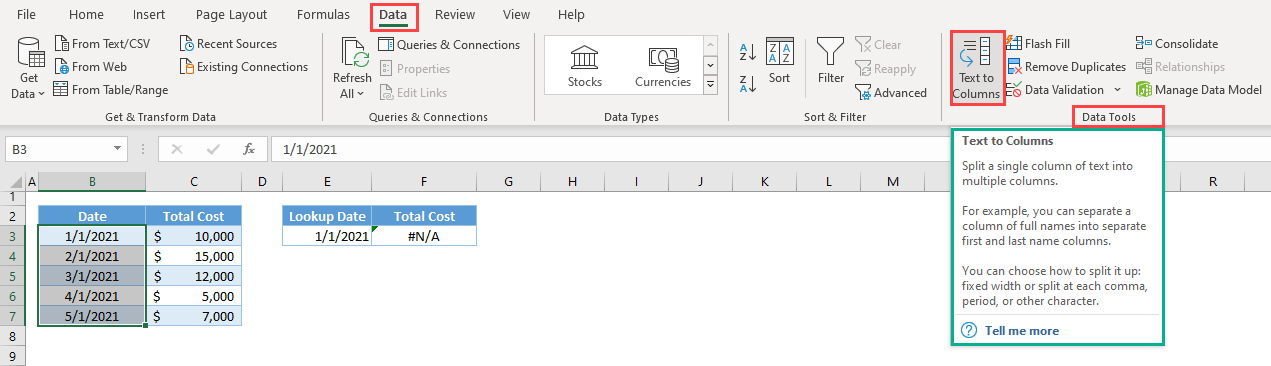1. In the popup window, select Delimited and click Next.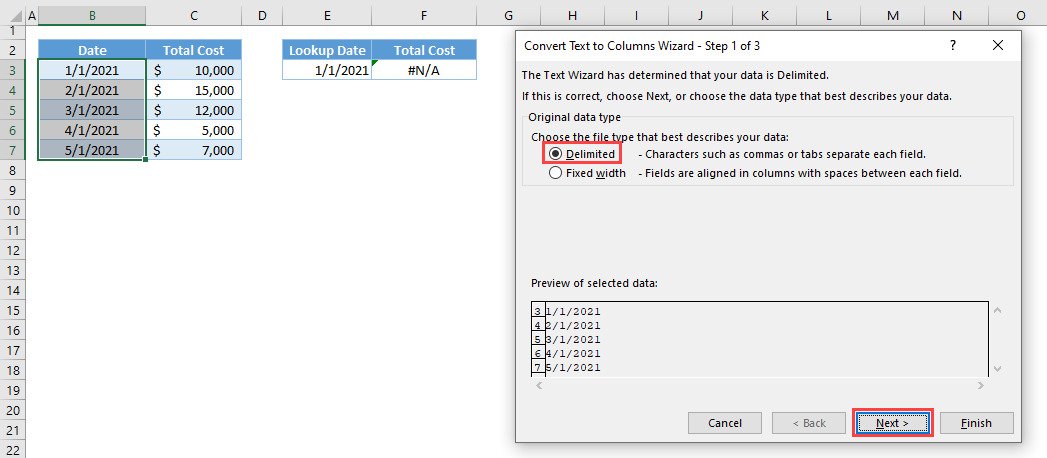1. In the next step, select Tab and click Next.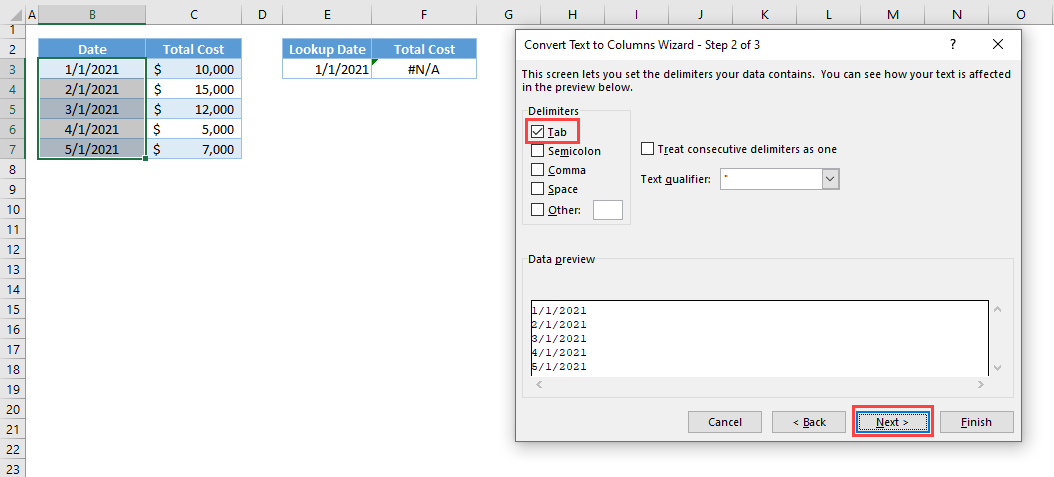1. In the last step, select Date and the format and click Finish.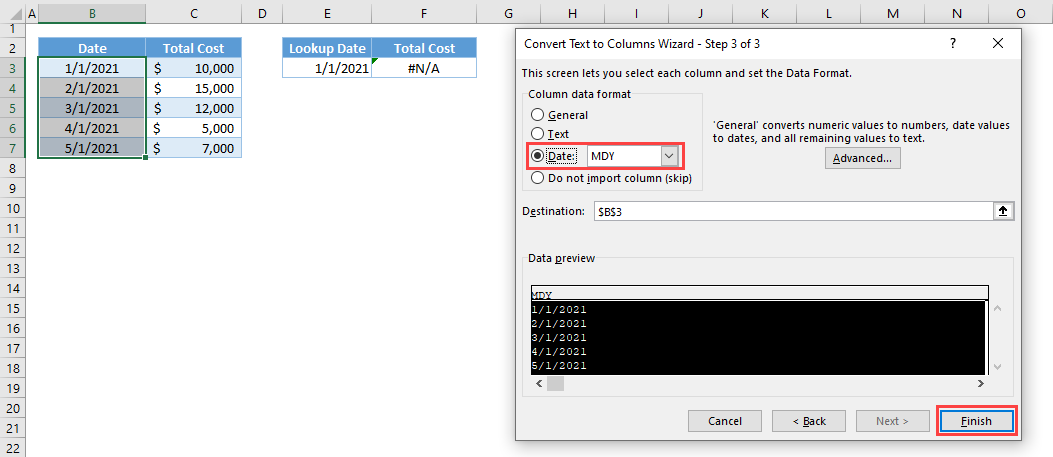1. The date will be converted into a date data type.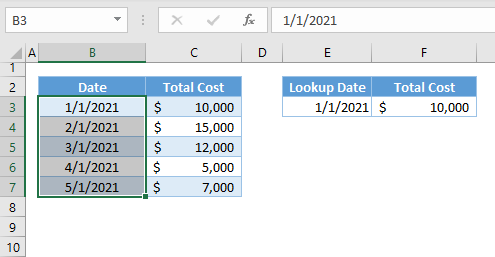### Excel Practice Worksheet

Practice Excel functions and formulas with our 100% free practice worksheets!